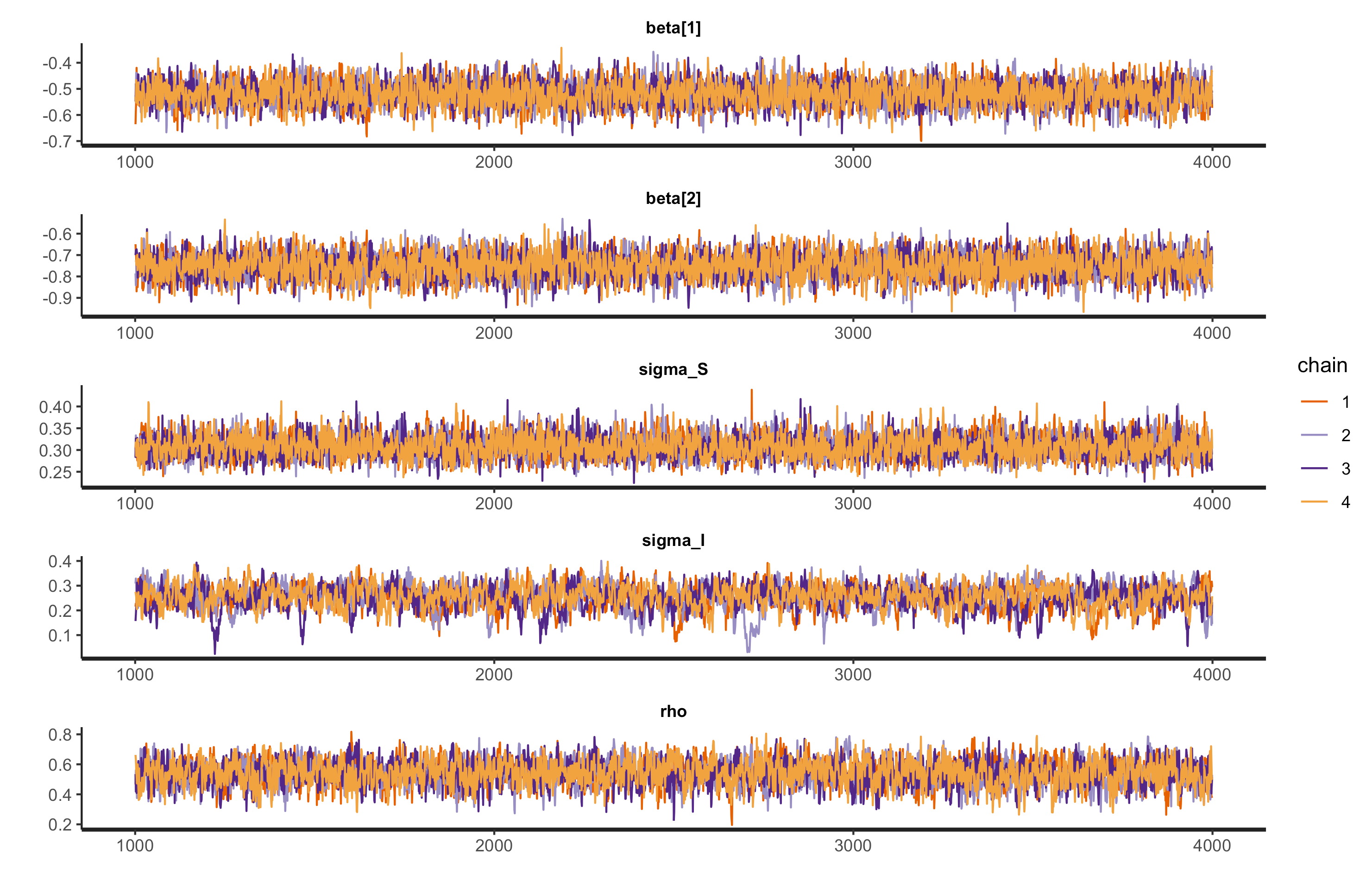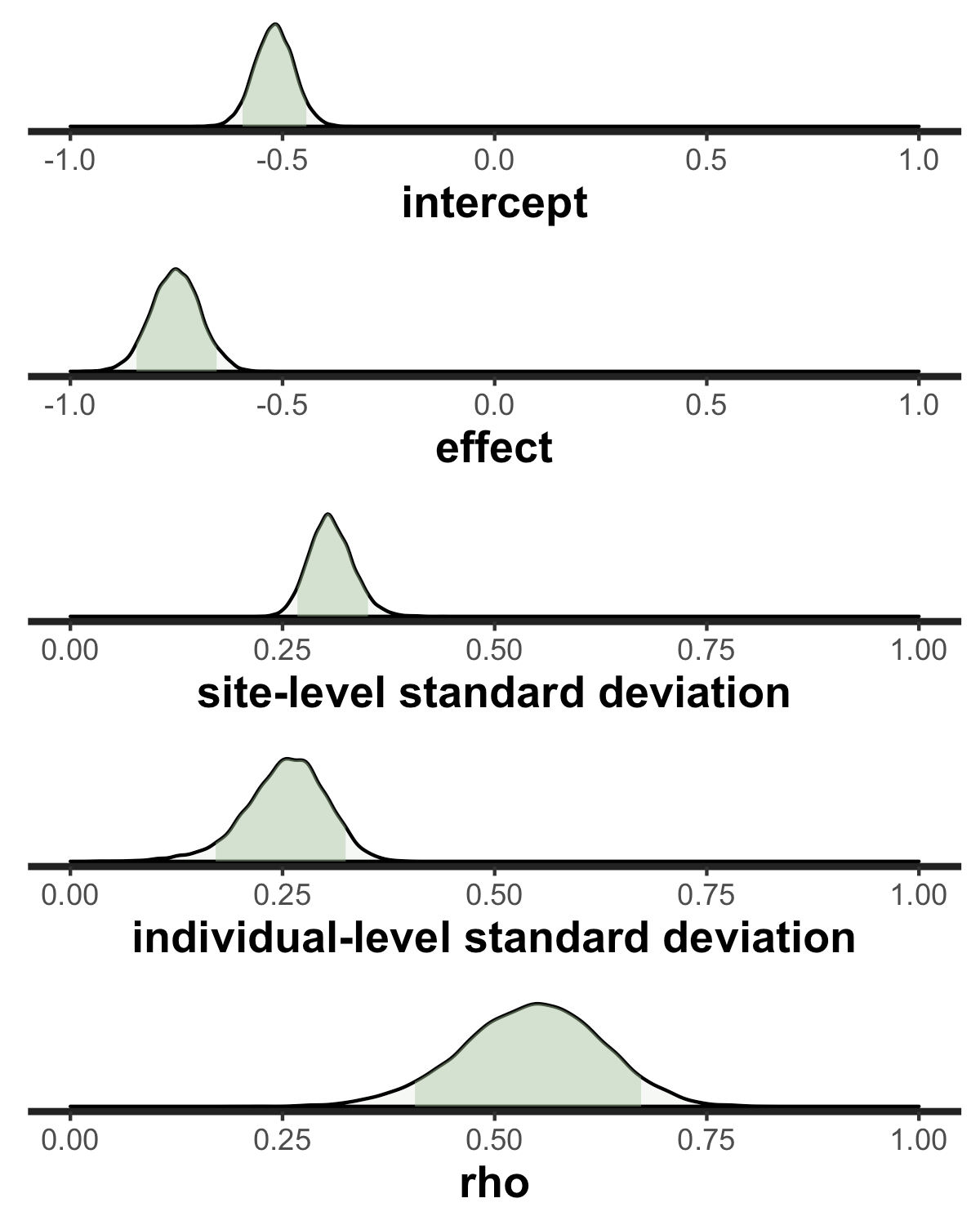Want to share your content on R-bloggers? click here if you have a blog, or here if you don't.

I am currently wrestling with how to analyze data from a stepped-wedge designed cluster randomized trial. A few factors make this analysis particularly interesting. First, we want to allow for the possibility that between-period site-level correlation will decrease (or decay) over time. Second, there is possibly additional clustering at the patient level since individual outcomes will be measured repeatedly over time. And third, given that these outcomes are binary, there are no obvious software tools that can handle generalized linear models with this particular variance structure we want to model. (If I have missed something obvious with respect to modeling options, please let me know.)

I introduced the project that is motivating all of this, the HAS-QOL study, when I provided simulations of the data generating process we are assuming for this analysis. And before that, I described (here and here) Bayesian models that can be used to analyze data with more complicated variance patterns, though all of those examples were based on continuous outcomes. Here, I am extending and combining those ideas to present an approach to estimating treatment effects in the context of HAS-QOL.

This post walks through the data generation process and describes a Bayesian model that addresses the challenges posed by the open cohort stepped-wedge study design.

The model

The process I use to simulate the data and then estimate to effects is based on a relatively straightforward logistic regression model with two random effects. To simplify things a bit, I intentionally make the assumption that there are no general time trends that affect that outcomes (though it would not be difficult to add in). In the logistic model, the log-odds (or logit) of a binary outcome is a linear function of predictors and random effects:

$\text{logit}(P(y_{ict}=1) = \beta_0 + \beta_1 I_{ct} + b_{ct} + b_i,$ where $$\text{logit}(P(y_{ict}=1))$$ is the log-odds for individual $$i$$ in cluster (or site) $$c$$ during time period $$t$$, and $$I_{ct}$$ is a treatment indicator for cluster $$c$$ during period $$t$$.

There are two random effects in this model. The first is a cluster-specific period random effect, $$b_{ct}$$ . For each cluster, there will actually be a vector of cluster effects $$\mathbf{b_c} = (b_{c0}, b_{c1},…,b_{c,T-1})$$, where $$\mathbf{b_c}\sim MVN(\mathbf{0}, \sigma_{b_c}^2\mathbf{R})$$, and $$\mathbf{R}$$ is

$\mathbf{R} = \left( \begin{matrix} 1 & \rho & \rho^2 & \cdots & \rho^{T-1} \\ \rho & 1 & \rho & \cdots & \rho^{T-2} \\ \rho^2 & \rho & 1 & \cdots & \rho^{T-3} \\ \vdots & \vdots & \vdots & \vdots & \vdots \\ \rho^{T-1} & \rho^{T-2} & \rho^{T-3} & \cdots & 1 \end{matrix} \right )$

The second random effect is the individual or patient-level random intercept $$b_i$$, where $$b_i \sim N(0,\sigma_{b_i}^2)$$. We could assume a more structured relationship for individual patients over time (such as a decaying correlation), but in this application, patients will not have sufficient measurements to properly estimate this.

In the model $$\beta_0$$ has the interpretation of the log-odds for the outcome when the the cluster is still in the control state and the cluster-period and individual effects are both 0. $$\beta_1$$ is the average treatment effect conditional on the random effects, and is reported as a log odds ratio.

Simulating the study data

I am going to generate a single data set based on this model. If you want more explanation of the code, this earlier post provides the details. The only real difference here is that I am generating an outcome that is a function of cluster-period effects, individual effects, and treatment status.

Site level data

There will be 24 sites followed for 12 periods ($$t=0$$ through $$t=11$$), and the stepped-wedge design includes 6 waves of 4 sites in each wave. The first wave will start at $$t=4$$, and a new wave will be added each period, so that the last wave starts at $$t=9$$.

library(simstudy)

dsite <- genData(24, id = "site")

dper <- addPeriods(dsite, nPeriods = 12, idvars = "site",
perName = "period")

dsw <- trtStepWedge(dper, "site", nWaves = 6, lenWaves = 1,
startPer = 4, perName = "period",
grpName = "Ict")

Correlated site-level effects

The average site-level effect is 0, the standard deviation of site averages is $$\sigma_{ct} = 0.3$$, and the correlation coefficient that will determine between-period within site correlation is $$\rho = 0.5$$. The correlation structure is “AR-1”, which means the between-period correlation decays over time (see definition of $$\mathbf{R}$$ above.)

siteDef <- defData(varname = "eff.mu", formula = 0,
dist = "nonrandom", id = "site")
siteDef <- defData(siteDef, varname = "eff.s2", formula = 0.3^2,
dist = "nonrandom")

dsw <- addCorGen(dsw, nvars = 12, idvar = "site", rho = 0.5,
corstr = "ar1", dist = "normal",
param1 = "eff.mu", param2 = "eff.s2",
cnames = "eff.st")

dsw <- dsw[, .(site, period, startTrt, Ict, eff.st)]

Patient level data

We are generating 20 patients per period for each site, so there will be a total of 5760 individuals ($$20\times24\times12$$). The individual level effect standard deviation $$\sigma_{b_i} = 0.3$$. Each of the patients will be followed until they die, which is a function of their health status over time, defined by the Markov process and its transition matrix defined below. (This was described in more detail in an earlier post.

dpat <- genCluster(dper, cLevelVar = "timeID",
numIndsVar = 20, level1ID = "id")

patDef <- defDataAdd(varname = "S0", formula = "0.4;0.4;0.2",
dist = "categorical")
patDef <- defDataAdd(patDef, varname = "eff.p",
formula = 0, variance = 0.3^2)

P <-t(matrix(c( 0.7, 0.2, 0.1, 0.0,
0.1, 0.3, 0.5, 0.1,
0.0, 0.1, 0.6, 0.3,
0.0, 0.0, 0.0, 1.0),
nrow = 4))

dpat <- addMarkov(dpat, transMat = P,
chainLen = 12, id = "id",
pername = "seq", start0lab = "S0",
trimvalue = 4)

dpat[, period := period + seq - 1]
dpat <- dpat[period < 12]

Individual outcomes

In this last step, the binary outcome $$y_{ict}$$ is generated based on treatment status and random effects. In this case, the treatment lowers the probability of $$Y=1$$.

dx <- merge(dpat, dsw, by = c("site","period"))
setkey(dx, id, period)

formula = "-0.5 - 0.8*Ict + eff.st + eff.p",
dist = "binary", link = "logit")

dx <- dx[, .(site, period, id, Ict, y)]

Here are the site-level averages over time. The light blue indicates periods in which a site is still in the control condition, and the dark blue shows the transition to the intervention condition. The lines, which are grouped by wave starting period, show the proportion of $$Y=1$$ for each period. You should be able to see the slight drop following entry into treatment.Estimating the treatment effect and variance components

Because none of the maximum likelihood methods implemented in R or SAS could estimate this specific variance structure using a mixed effects logistic regression model, I am fitting a Bayesian model using RStan and Stan, which requires a set of model definitions.

This model specification is actually quite similar to the model I estimated earlier, except of course the outcome distribution is logistic rather than continuous. Another major change is the use of a “non-centered” parameterization, which actually reduced estimation times from hours to minutes (more precisely, about 12 hours to about 30 minutes). This reparameterization requires a Cholesky decomposition of the variance-covariance matrix $$\Sigma$$. One additional limitation is that proper convergence of the MCMC chains seems to require a limited prior on $$\rho$$, so that $$\rho \sim U(0,1)$$ rather than $$\rho \sim U(-1,1)$$.

This particular code needs to be saved externally, and I have created a file named binary sw - ar ind effect - non-central.stan. This file is subsequently referenced in the call to RStan.

data {
int<lower=1> I;              // number of unique individuals
int<lower=1> N;              // number of records
int<lower=1> K;              // number of predictors
int<lower=1> J;              // number of sites
int<lower=0> T;              // number of periods
int<lower=1,upper=I> ii[N];  // id for individual
int<lower=1,upper=J> jj[N];  // group for individual
int<lower=1,upper=T> tt[N];  // period of indidvidual
matrix[N, K] x;              // matrix of predictors
int<lower=0,upper=1> y[N];   // vector of binary outcomes
}

parameters {
vector[K] beta;              // model fixed effects
real<lower=0> sigma_S;       // site variance (sd)
real<lower=0,upper=1> rho;   // correlation
real<lower=0> sigma_I;       // individual level varianc (sd)

// non-centered paramerization

vector[T] z_ran_S[J];   // site level random effects (by period)
vector[I] z_ran_I;      // individual level random effects
}

transformed parameters {

cov_matrix[T] Sigma;
matrix[T, T] L;         // for non-central parameterization
vector[I] ran_I;        // individual level random effects
vector[T] ran_S[J];     // site level random effects (by period)
vector[N] yloghat;

// Random effects with exchangeable correlation

real sigma_S2 = sigma_S^2;

for (j in 1:T)
for (k in 1:T)
Sigma[j,k] = sigma_S2 * pow(rho,abs(j-k));

// for non-centered parameterization

L = cholesky_decompose(Sigma);

for(j in 1:J)
ran_S[j] = L * z_ran_S[j];

ran_I = sigma_I * z_ran_I;

// defining mean on log-odds scale

for (i in 1:N)
yloghat[i] = x[i]*beta + ran_S[jj[i], tt[i]] + ran_I[ii[i]];

}

model {

sigma_I ~ exponential(0.25);
sigma_S ~ exponential(0.25);

rho ~ uniform(0, 1);

for(j in 1:J) {
z_ran_S[j] ~ std_normal();
}

z_ran_I ~ std_normal();

y ~ bernoulli_logit(yloghat);

}

Set up the data and call stan from R

Just for completeness, I am providing the code that shows the interface between R and Stan using RStan. The data needs to be sent to Stan as a list of data elements, which here is called testdat. For the estimation of the posterior probabilities, I am specifying 4 chains of 4000 iterations each, which includes 1000 warm-up iterations. I specified “adapt_delta = 0.90” to reduce the step-size a bit (default is 0.80); this slows things down a bit, but improves stability.

As I mentioned earlier, with this data set (and rather large number of effects to estimate), the running time is between 30 and 45 minutes. One of the downsides of this particular Bayesian approach is that it wouldn’t really be practical to do any kind of sample size estimate.

x <- as.matrix(dx[ ,.(1, Ict)])
I <- dx[, length(unique(id))]
N <- nrow(x)
K <- ncol(x)
J <- dx[, length(unique(site))]
T <- dx[, length(unique(period))]
ii <- dx[, id]
jj <- dx[, site]
tt <- dx[, period] + 1
y <- dx[, y]

testdat <- list(I=I, N=N, K=K, J=J, T=T, ii=ii, jj=jj, tt=tt, x=x, y=y)

library(rstan)
options(mc.cores = parallel::detectCores())

rt <- stanc("binary sw - ar ind effect - non-central.stan")
sm <- stan_model(stanc_ret = rt, verbose=FALSE)

fit.ar1 <- sampling(sm, data=testdat,
iter = 4000, warmup = 1000,
max_treedepth = 15),
chains = 4)

Diagnostics

After running the MCMC process to generate the probability distributions, the trace plots show that the mixing is quite adequate for the chains.

plot(fit.ar1, plotfun = "trace", pars = pars,
inc_warmup = FALSE, ncol = 1)Extracting results

If we take a look at the posterior probability distributions, we can see that they contain the original values used to generate the data - so at least in this case, the model seems to model the original data generation process quite well.

pars <- c("beta", "sigma_S","sigma_I","rho")
summary(fit.ar1, pars = pars, probs = c(0.025, 0.975))\$summary
##           mean  se_mean     sd   2.5%  97.5% n_eff Rhat
## beta -0.519 0.000687 0.0459 -0.609 -0.428  4470    1
## beta -0.751 0.000844 0.0573 -0.864 -0.638  4618    1
## sigma_S  0.307 0.000394 0.0256  0.260  0.362  4223    1
## sigma_I  0.254 0.001548 0.0476  0.148  0.337   945    1
## rho      0.544 0.001594 0.0812  0.376  0.698  2599    1One thing that is not working so well is my attempt to compare different models. For example, I might want to fit another model that does not assume between-period correlations decay and compare it to the current model. Previously, I used the bridgesampling package for the comparisons, but it does not seem to be able to accommodate these models. I will continue to explore the options more model comparison and will report back if I find something promising.

This study is supported by the National Institutes of Health National Institute on Aging R61AG061904. The views expressed are those of the author and do not necessarily represent the official position of the funding organizations.